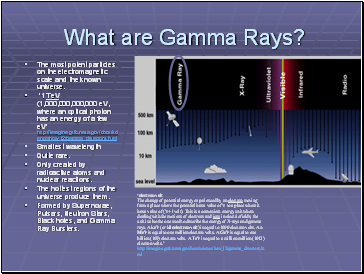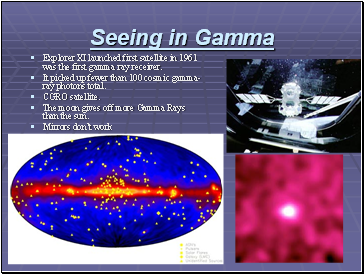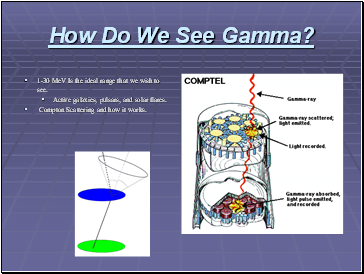# Gamma-RaysPage 1

#### WATCH ALL SLIDES

Slide 1Project Gamma

By Wylie Ballinger and Sam Russell

Slide 2## What are Gamma Rays?

The most potent particles on the electromagnetic scale and the known universe.

‘1 TeV (1,000,000,000,000 eV, where an optical photon has an energy of a few eV’ http://imagine.gsfc.nasa.gov/docs/science/how_l2/gamma_detectors.html

Smallest wavelength

Quite rare.

Only created by radioactive atoms and nuclear reactions.

The hottest regions of the universe produce them.

Formed by Supernovae, Pulsars, Neutron Stars, Black holes, and Gamma Ray Bursters.

‘electron volt

The change of potential energy experienced by an electron moving from a place where the potential has a value of V to a place where it has a value of (V+1 volt). This is a convenient energy unit when dealing with the motions of electrons and ions in electric fields; the unit is also the one used to describe the energy of X-rays and gamma rays. A keV (or kiloelectron volt) is equal to 1000 electron volts. An MeV is equal to one million electron volts. A GeV is equal to one billion (109) electron volts. A TeV is equal to a million million (1012) electron volts.’ http://imagine.gsfc.nasa.gov/docs/science/how_l2/gamma_detectors.html

Slide 3## Seeing in Gamma

Explorer XI launched first satellite in 1961 was the first gamma ray receiver.

It picked up fewer than 100 cosmic gamma-ray photons total.

CGRO satellite.

The moon gives off more Gamma Rays than the sun.

Mirrors don’t work

Slide 4## How Do We See Gamma?

1-30 MeV Is the ideal range that we wish to see.

Active galaxies, pulsars, and solar flares.

Compton Scattering and how it works.

Slide 5How Do We See in Gamma? Continued…

I’m a visual person…

Slide 6## So How Does That Work Again?

Crystal Scintillators

Crystals that emit low energy light (usually visible) when hit by high energy light such as gamma radiation.

Pair Production

The physical process whereby a gamma-ray photon, usually through an interaction with the electromagnetic field of a nucleus, produces an electron and an anti-electron (positron). The original photon no longer exists, its energy having gone to the two resulting particles. The inverse process, pair annihilation, creates two gamma-ray photons from the mutual destruction of an electron/positron pair. http://imagine.gsfc.nasa.gov/docs/dict_jp.html#pair_production

Go to page:
1  2  3